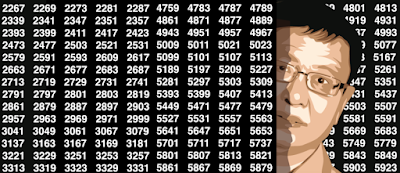## Wednesday, December 27, 2017

### A Simple Introduction to Complex Stochastic Processes

Stochastic processes have many applications, including in finance and physics. It is an interesting model to represent many phenomena. Unfortunately the theory behind it is very difficult, making it accessible to a few 'elite' data scientists, and not popular in business contexts.
One of the most simple examples is a random walk, and indeed easy to understand with no mathematical background. However, time-continuous stochastic processes are always defined and studied using advanced and abstract mathematical tools such as measure theory, martingales, and filtration. If you wanted to learn about this topic, get a deep understanding on how they work, but were deterred after reading the first few pages of any textbook on the subject due to jargon and arcane theories, here is your chance to really understand how it works.
Rather than making it a topic of interest to post-graduate scientists only, here I make it accessible to everyone, barely using any maths in my explanations besides the central limit theorem. In short, if you are a biologist, a journalist, a business executive, a student or an economist with no statistical knowledge beyond Stats 101, you will be able to get a deep understanding of the mechanics of complex stochastic processes, after reading this article. The focus is on using applied concepts that everyone is familiar with, rather than mathematical abstraction.
My general philosophy is that powerful statistical modeling and machine learning can be done with simple techniques, understood by the layman, as illustrated in my article on machine learning without mathematics or advanced machine learning with basic excel
1. Construction of Time-Continuous Stochastic Processes: Brownian Motion
Probably the most basic stochastic process is a random walk where the time is discrete. The process is defined by X(t+1) equal to X(t) + 1 with probability 0.5, and to X(t) - 1 with probability 0.5. It constitutes an infinite sequence of auto-correlated random variables indexed by time. For instance, it can represent the daily logarithm of stock prices, varying under market-neutral conditions. If we start at t = 0 with X(0) = 0, and if we define U(t) as a random variable taking the value +1 with probability 0.5, and -1 with probability 0.5, then X(n) = U(1) + ... + U(n).  Here we assume that the variables U(t) are independent and with the same distribution. Note that X(n) is a random variable taking integer values between -n and +n.
Five simulations of a Brownian motion  (x-axis is the time t, u-axis is Z(t)
What happens if we change the time scale (x-axis) from daily to hourly, or to every millisecond? We then also need to re-scale the values (y-axis) appropriately, otherwise the process exhibits massive oscillations (from -n to +n) in very short time periods. At the limit, if we consider infinitesimal time increments, the process becomes a continuous one. Much of the complex mathematics needed to define these continuous processes do no more than finding the correct re-scaling of the y-axis, to make the limiting process meaningful.